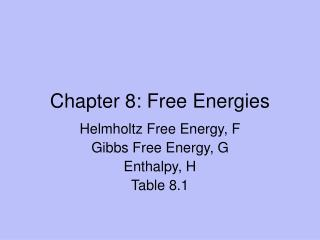# Chapter 8: Free Energies - PowerPoint PPT PresentationDownload PresentationChapter 8: Free Energies

Chapter 8: Free Energies
Download Presentation## Chapter 8: Free Energies

- - - - - - - - - - - - - - - - - - - - - - - - - - - E N D - - - - - - - - - - - - - - - - - - - - - - - - - - -
##### Presentation Transcript

1. Chapter 8: Free Energies Helmholtz Free Energy, F Gibbs Free Energy, G Enthalpy, H Table 8.1

2. I. Equilibrium – Ch. 7 • In Ch. 7, we started with differences in T, p or μ between two subsystems. • The total system was isolated from the surroundings but U, V or N were exchanged (the variables) between the subsystems. • As a result, equilibrium was associated with maximum S(U, V, N).

3. Equilibrium – Ch. 8 • Now consider a sealed test tube in which V and N are constant. This test tube is in a constant water bath, so heat (= energy) is exchanged to maintain constant T. (Confirm using First Law) • When T, V and N are the variables, then equilibrium is associated with minimum F(T, V, N): dF ≤ 0. Eqns 8.1 – 8.6

4. Natural Variables • There is a distinction between “variables” and “natural variables” of thermodynamic functions (TF). • When a TF is expressed in terms of its natural variables, then the TF can be used to find equilibrium. I.e. the Extremum Principle is justified. • Max S(U, V, N) but not S(T, V, N) • Min G(T, p, N) but not G(U, V, N)

5. Thermodynamic Function, F • F(T, V, N) = U – TS is called the Helmhotz Free Energy. • Then dF = dU – T dS ≤ 0 and equilibrium is associated with min U and max S at constant T. • dF = (δF/δT)V,NdT + (δF/δV)T,NdV + Σ(δF/δNj)V,T,Ni dNj = - S dT – p dV + Σμj dNj • Examples 8.1, 8.2

6. Thermodynamic Function, H • H(S, p, N) = U + pV is called Enthalpy. • dH = T dS + V dp + Σμj dNj Eqn 8.22 • ΔH values are experimentally accessible (calorimetry).

7. Thermodynamic Function, G • G(T, p, N) = H –TS is called the Gibbs Free Energy. • dG = -S dT + V dp + Σμj dNjEqn 8.25 • Then dG = dH – T dS ≤ 0 and equilibrium is associated with min H and max S at constant T. • Note Table 8.1

8. II. More Applications • Ch 7 Tools • In an adiabatic process, w  ΔU (dU = δw) • Using the First Law, q  ΔS (δq = dS/T) (Carnot Cycle) • Ch 8 Tools • Heat Capacity • Cycles

9. Heat Capacity  ΔU, ΔS  ΔF • q = heat αΔT and the proportionality constant is specific heat (J/g-K) or heat capacity (C, J/mol-K) • Note C may = f(T); Table 8.2 • When ΔV = 0 (bomb calorimeter), dU = δq. Then CV = (δq/dT)V = (∂U/∂T)V = T(∂S/∂T)V • dU = CV dT and dS = CV dT /T Eqn 8.31 • Ex 8.5, 8.6

10. Heat Capacity  ΔH, ΔS  ΔG • When Δp = 0, then dH = δq. Then Cp = (δq/dT)p = (∂H/∂T)p = T(∂S/∂T)p • dH = Cp dT and dS = Cp dT /T Eqn 8.34

11. Cycles (ΔTF = 0)  non-measurables • Determine ΔH for a phase change not at equilibrium. • Internal combustion engine • Adiabatic gas expansion • Otto Cycle (note compression ratio)